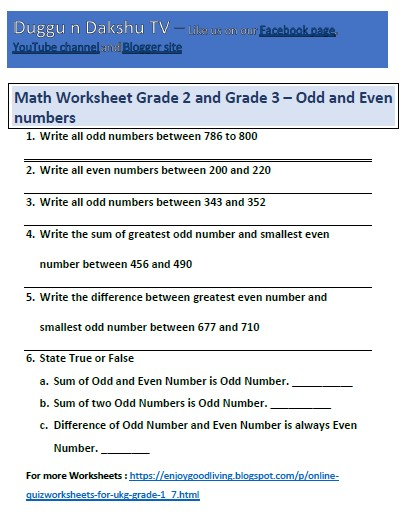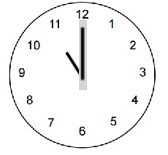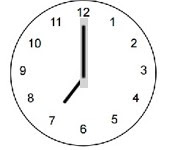# Odd and Even Number Exercises for Class 2 and Class 3 Students1. Write all odd numbers between 786 to 800
2. Write all even numbers between 200 and 220
3. Write all odd numbers between 343 and 352
4. Write the sum of greatest odd number and smallest even number between 456 and 490
5. Write the difference between greatest even number and smallest odd number between 677 and 710
6. State True or False
• Sum of Odd and Even Number is Odd Number. __________
• Sum of two Odd Numbers is Odd Number. __________
• Difference of Odd Number and Even Number is always Even Number. ________

# Math Worksheet LKG, UKG, Grade 1 and Grade2 – Days of Week

of Week

Arrange the days of week in right order:

 Days Right Order Sunday Monday Monday _______________ Thursday _______________ Saturday _______________ Tuesday _______________ Friday _______________ Wednesday _______________
 How many days are there in a week? _______________ days Complete the pattern Monday Tuesday ____________ What day comes after Friday? _______________ Complete the pattern Tuesday Thursday ____________ What day comes before Monday? _______________ If today is Saturday, what day is tomorrow? _______________ What day comes between Monday and Wednesday? _______________ If today is Friday, what day was yesterday? _______________ If today is Monday, what day is day after tomorrow? _______________ If today is Wednesday, what day is day before yesterday? _______________

# Math Worksheet LKG, UKG, Grade 1 and Grade2 – Write missing number in addition by Crossing and Counting Objects – Number up to 10

missing number in addition by Crossing and Counting Objects – Number up to 10

# Math Worksheet – UKG, Grade 1 and Grade 2 – Clock Time

Time

## Write Clock Time________ o’clock________ o’clock________ o’clock________ o’clock________ o’clock________ o’clock

# Math Worksheet UKG, Grade 1 and Grade2 – Write missing number in addition – Number up to 100

missing number in addition – Number up to 100

## Write missing number

 20 + ______ = 70 56 + ______ = 80 ______ + 45 = 90 ______ + 62 = 78 34 + ______ = 64 12 + ______ = 48 ______ + 91 = 91 ______ + 34 = 82 1 + ______   = 70 1 + ______   = 22 ______ + 2   = 51 ______ + 2   = 32 ______ + 85 = 89 ______ + 54 = 61 71 + ______ = 79 49 + ______ = 54 ______ + 11 = 74 ______ + 13 = 95 37 + ______   = 74 48 + ______ = 96

# Math Worksheet UKG, Grade 1 and Grade 2 – ADDITION AND SUBTRACTION – 10 MORE or 10 LESS – Number up to 100

Math Worksheet UKG, Grade 1 and Grade 2 – ADDITION AND SUBTRACTION – 10 MORE or 10 LESS – Number up to 1000

## 10 more or 10 less

 90 + 10 = ________ 80 – 10 = ________ 43 + 10 = ________ 71 – 10 = ________ 57 + 10 = ________ 36 – 10 = ________ 81 + 10 = ________ 64 – 10 = ________ 75 + 10 = ________ 59 – 10 = ________

 10 more 10 less 78 ______ _______ 98 62 ______ _______ 100 44 ______ _______ 81 96 ______ _______ 52 39 ______ _______ 17